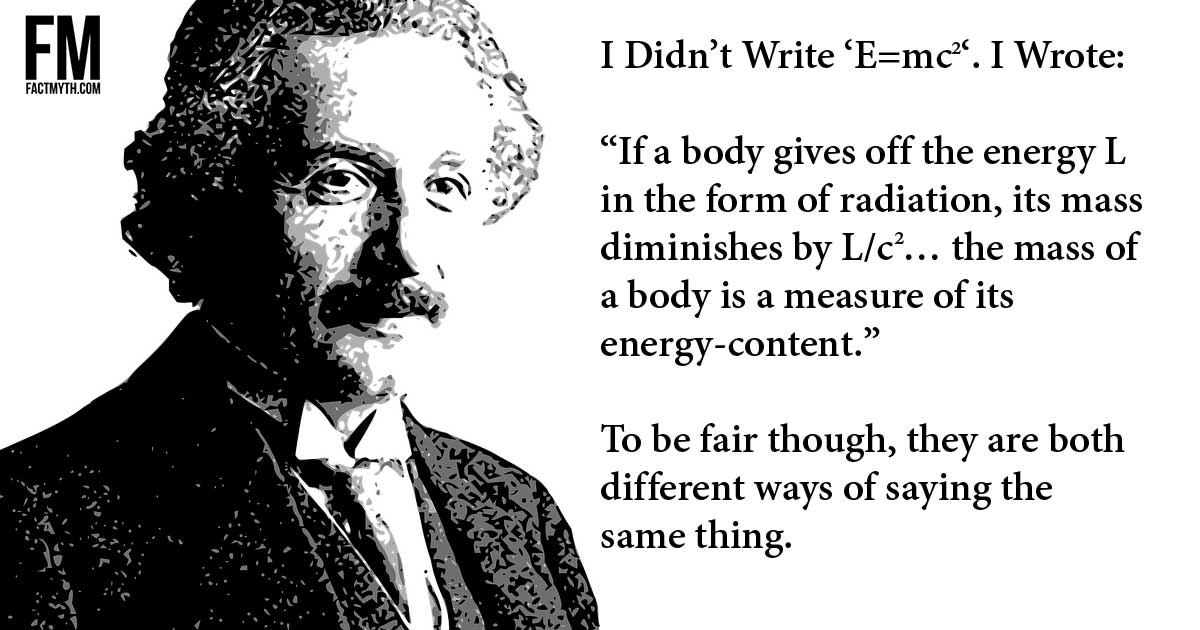Myth

Einstein wrote down the famous physics equation ‘E=mc2‘ in his 1905 paper "DOES THE INERTIA OF A BODY DEPEND UPON ITS ENERGY-CONTENT?"

## Did Einstein Actually Write E=mc2?

Einstein’s 1905 paper on mass-energy equivalence doesn’t actually say ‘E=mc2‘ it says, roughly, ‘m=L/c2‘.

With the above in mind, Einstein notably did write the equation as ‘E=mc2‘ in the first issue of Science Illustrated in 1946 in the article “E=mc²: The Most Urgent Problem of Our Time” (as one reader pointed out).

Thus, one shouldn’t take the above to mean that Einstein never said ‘E=mc2‘, but only to mean that he didn’t say it in his famous paper “DOES THE INERTIA OF A BODY DEPEND UPON ITS ENERGY-CONTENT?

## What Does Einstein’s Paper Say?

In his paper however the famous physics equation never appears. Instead, Einstein wrote (translated from German):

“If a body gives off the energy L in the form of radiation, its mass diminishes by L/c2… the mass of a body is a measure of its energy-content”.

The L stands for “Lagrangian,” a type of energy, so (paraphrasing) it would be m=E/c2. This was later translated to an easier meme, and equally correctly stated as E=mc2.

Einstein did not write E=mc2 and the exact translation of his exact words depends on how good your German is, or which translation you are reading.

See two examples of translations:

1. DOES THE INERTIA OF A BODY DEPEND UPON ITS ENERGY-CONTENT?” – Translation 1
2. DOES THE INERTIA OF A BODY DEPEND UPON ITS ENERGY-CONTENT?” – Translation 2

## Other Ways to Write the Mass-energy Equivalence Equation

The short form version of the mass-energy equivalence equation (the one that doesn’t factor in motion) can be written in several of ways (including E=mc2, m=E/c2, and c2= E/m). All of these are actually just shorthand for more complex equations that do factor in motion.

E=mc2 or it’s inversion m=E/c(when paired with everything else we know) represents a concept which opens the door for us to understand the true nature of particles, spacetime, and the universe. This is why it’s physics #1 meme.

## Life After 26

Einstein published his miracle year papers at the age of 26 in 1905, but he worked on the concept of relativity for the rest of his life and many others worked on it as well.

How Einstein Discovered E=mc2.

FACT: “DOES THE INERTIA OF A BODY DEPEND UPON ITS ENERGY-CONTENT?” By A. Einstein was published in German on September 27, 1905 (published as Ist die Tr¨agheit eines K¨orpers von seinem Energiegehalt abh¨angig? in Annalen der Physik. 18:639, 1905). It later appeared in the book The Principle of Relativity, published in 1923 by Methuen and Company, Ltd. of London.

Conclusion

Einstein’s paper doesn’t actually say ‘E=mc2‘ it says, roughly, ‘m=L/c2‘. To be fair though, that is just another way to say the same thing. To be even more fair, Eisenstein later used ‘E=mc2‘ himself.

References

"Einstein Wrote ‘E=mc^2’ in His Famous Paper" is tagged with: Albert Einstein, RelativityFranklin Williams on
Doesn't beleive this myth.

Einstein actually wrote E=m/c^2, saying that the weight of the energy in an object is equal to Mass(Measured Mass) divided by the constant of the speed of light. This is used to find the rest mass of an object(mass of an object at absolute zero[0* Kelvin]). Einstein did not write E=mc^2 or m=L/c^2, he wrote E=m/c^2.Henry K.O. Norman on

Stubborn myth, but really one of semantics